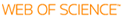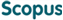### BROWSE

#### Related ResearcherJang, Bongsoo
Computational Mathematical Science Lab (CMS Lab)
Research Interests
• Numerical analysis, dynamics, fractional systems, data sciences

Shifted Jacobi spectral-Galerkin method for solving fractional order initial value problems

Cited 0 times inCited 0 times inTitle
Shifted Jacobi spectral-Galerkin method for solving fractional order initial value problems
Author
Issue Date
2020-12
Publisher
ELSEVIER
Citation
JOURNAL OF COMPUTATIONAL AND APPLIED MATHEMATICS, v.380, pp.112988
Abstract
In this paper, the shifted Jacobi spectral-Galerkin method is introduced to deal with fractional order initial value problems (FIVPs). In the proposed method, the exact solution of the FIVP is approximated by using shifted Jacobi polynomials on each subinterval of the total time. The main advantage of the proposed method is that the rate of convergence in L-2-norm depends on the local smoothness of solution. This enables the proposed method to work for nonsmooth solutions. The second merit is that the flexibility between the length of sub-interval and the degree of polynomial significantly enhances the numerical accuracy. We derive the spectral-Galerkin approximation formula for the Volterra integral equation of the second kind which is equivalent to the FIVP. Error analysis in L-2-norm for the case alpha = beta = 0 in shifted Jacobi polynomials is provided and it justifies the spectral rate of convergence with respect to both the degree and the length of the subinterval when the source function is Lipschitz continuous in the second argument. Numerical illustrations for multi-order, linear, and nonlinear FIVPs are demonstrated to confirm the convergence rate. (C) 2020 Elsevier B.V. All rights reserved.
URI
https://scholarworks.unist.ac.kr/handle/201301/32310
URL
https://www.sciencedirect.com/science/article/pii/S037704272030279X
DOI
10.1016/j.cam.2020.112988
ISSN
0377-0427
Appears in Collections:
PHY_Journal Papers
Files in This Item:
There are no files associated with this item.